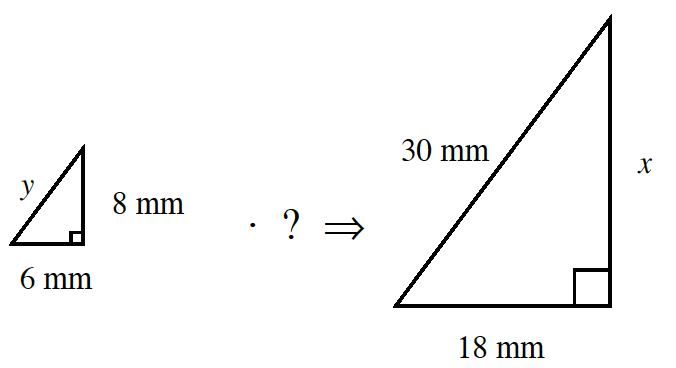### Home > CC2MN > Chapter 6 Unit 6A > Lesson CC3: 6.2.5 > Problem6-92

6-92.

Sketch the two similar triangles at right on your own paper. Find the scale factor and the missing side lengths.Since the scale factor applies to all sides of the shape equally, the two triangles share a common side ratio.

Based on the triangles above, use the given side lengths to find the scale factor ratio between the two triangles.
While each triangle is missing a certain side length, do you notice a pair of sides that are both apparent?

Use the scale factor you have solved for to determine the missing side lengths by either multiplying or dividing.

Scale Factor: $3:1$
Now try solving for $y$ and $x$.